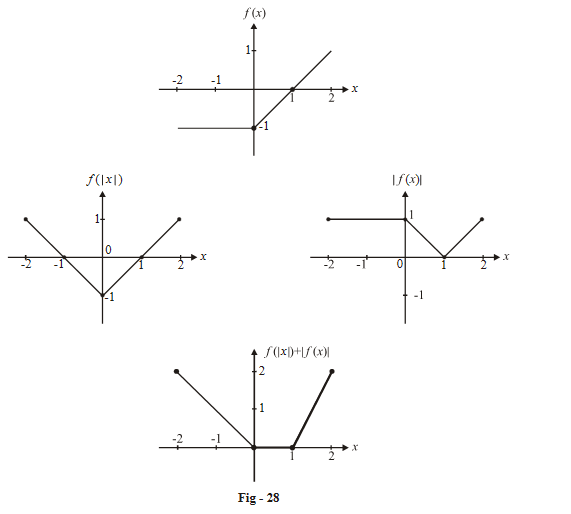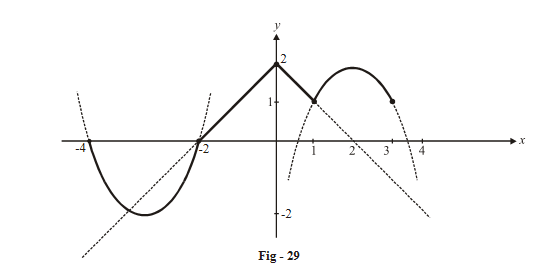# Examples On Differentiability Set-2

Go back to  'LCD'

## Differentiability examples

Example - 21

Let f\left( x \right) = \left\{ {\begin{align}{}{ - 1,} {\;\;\;\;\; - 2 \le x \le 0}\\{x - 1,} {\;\;\;\;\;0 < x \le 2}\end{align}} \right\}{\rm{and }}g\left( x \right) = f\left( {\left| x \right|} \right) + \left| {f\left( x \right)} \right|.

Evaluate the continuity and differentiability of $$g\left( x \right)$$  in the interval [–2, 2] by drawing the graph.

Solution:    From the graph of   $$f\left( x \right)$$ , we can easily derive the graphs of $$f\left( {\left| x \right|} \right)\,\,{\rm{and}}\,\left| {f\left( x \right)} \right|,$$  and add them point by point to get the graph of $$g\left( x \right)$$ .Verify for yourself the result of the addition of the two graphs.

It is obvious from the resultant graph that $$g\left( x \right)$$   is continuous everywhere but non-differentiable at x = 0 and  x =1

Example - 22

If  f\left( x \right) = \left\{ {\begin{align}&{2{x^2} + 12x + 16,} {\;\;\;\;\; - 4 \le x \le - 2}\\&{2 - \left| x \right|\,\,\,\,\,\,\,\,\,\,\,\,\,\,\,\,\,\,,} {\;\;\;\;\; - 2 < x \le 1}\\&{4x - {x^2} - 2\,\,\,\,\,\,\,,} {\;\;\;\;\;1 < x \le 3}\end{align}} \right\}, evaluate the continuity and differentiability of f(x)

Solution:    This graph will have 3 different segments which can easily be plotted as shown in below (you are urged to carry out the plotting on your own):We first draw all the three functions’ graphs on the axis using dotted curves and then darken the relevant portions as specified in the piecewise domain.

For example, we selected only that segment of   $$2{x^2} + 12x + 16$$  for which $$x \in \left[ { - 4, - 2} \right]$$   and so on.

It is clear from the graph that f (x) is continuous in its entire domain [–4, 3] but is non-differentiable at 3 points x = –2, 0 ,1.

The evaluation of left and right derivatives on each of these three points is left to you as an exercise. The results are:

\begin{align} \boxed{x = - 2} \;{\quad \text{LHD}} &= 4 \;\,{\qquad\text{RHD}} = - 1 \\ \boxed{x = 0} {\qquad \text{LHD}} &= 1 {\;\;\qquad\text{RHD}} = - 1 \\ \boxed{x = 1} {\qquad\text{LHD}} & = -1\;\;\;{\quad\text{RHD}} = 2 \\ \end{align}